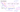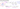## Logistic Regression: Decision Boundary

In order to get our discrete 0 or 1 classification, we can translate the output of the hypothesis function as follows:

\begin{aligned} h_\theta(x) \geq 0.5 \space \Rightarrow \space y = 1 \\ h_\theta(x) < 0.5 \space \Rightarrow \space y = 0\end{aligned}

Recall that for the logistic function $g(z) = \dfrac{1}{1 + e^{-z}}$, we have $g(z) \ge 0.5$ when $z \ge 0$.

Therefore, $h_\theta(x) = g(\theta^T x) \geq 0.5 \space$ when $\space\theta^T x \geq 0$

\begin{aligned} \theta^T x \geq 0 \space \Rightarrow \space y = 1 \\ \theta^T x < 0 \space \Rightarrow \space y = 0 \end{aligned}

The decision boundary is the line that separates the area where y = 0 and where y = 1. It is created by our hypothesis function using the equation:

\begin{aligned} h_\theta(x) = 0.5 \\ or, \space \theta^T x = 0 \end{aligned}The input $(\theta^Tx)$ to the sigmoid function $g(z)$ doesn't need to be linear, and could be a function that describes a circle (e.g. $z = \theta_0 + \theta_1 x_1^2 +\theta_2 x_2^2$) or any other shape to fit our data.